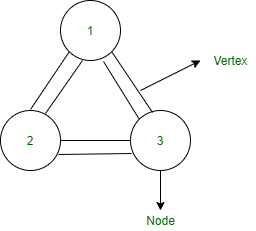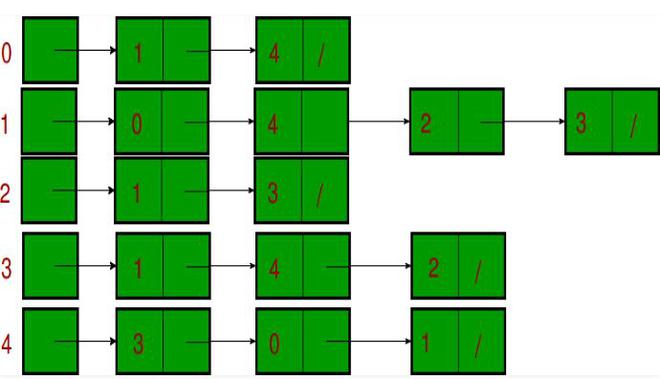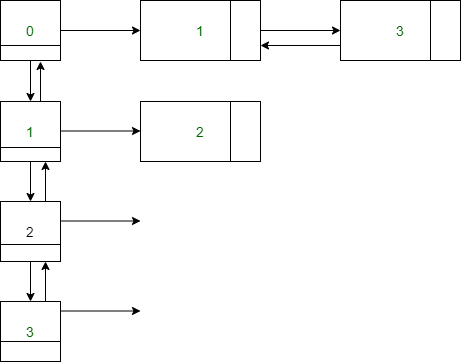Skip to content
Related Articles
Java Program to Represent Graphs Using Linked List
• Difficulty Level : Easy
• Last Updated : 08 May, 2021

Data structures are divided into two categories Linear data structures and Non-Linear data structures. The major disadvantage of the linear data structure is we cannot arrange the data of linear data structure in hierarchy manner that’s why in the computer field we use Non-Linear data structures. The most commonly used Non-Linear data structure is Graphs and Trees both data structures can be implemented using Linear data structures. In this article, we will discuss how to represent Graphs using Linked List.

Graphs consist of a finite set of vertices(or nodes) and a set of edges that connect a pair of nodes. Graphs are represented in two different ways. One method is using adjacency List representation and the second is adjacency matrix representation. Adjacency List representation is mostly used because of dynamic memory allocation using list representation.

Graphs are of two types:

• Directed Graphs: In this arrangement of graphs, every node is directed to one vertex is called directed Graphs.
• Undirected Graphs: In this arrangement of graphs, two nodes are connected using bi-direction vertex is called undirected graphs.

Undirected Graphs representation: Maximum number of a vertex in the undirected graphs is n*(n-1) where n is the total number of nodes present in the Undirected Graphs.LinkedList Representation of undirected Graphs is as follows:

In undirected graphs, two nodes are connected in bi-direction vertex. We can use both Array List and Linked List collections to represent the undirected graphs. In Linked List the Manipulation of the data is faster than the Array List because Array List internally used the dynamic array to store the data whereas Linked List used Doubly Linked List that is faster in the operation of manipulation but not in accessing the elements.Implementation: Here we will be discussing out both types of graphs in order to implement the same.

Example 1

## Java

 `// Java Program to Implement the Unidirectional Graph``// Using Linked List`` ` `// Importing required classes from packages``import` `java.io.*;``import` `java.util.*;`` ` `// Main class``class` `GFG {`` ` `    ``// Method 1``    ``// To make pair of nodes``    ``static` `void``    ``addEdge(LinkedList > Adj, ``int` `u,``            ``int` `v)``    ``{``        ``// Creating bi-directional vertex``        ``Adj.get(u).add(v);``        ``Adj.get(v).add(u);``    ``}`` ` `    ``// Method 2``    ``// To print the adjaceny list``    ``static` `void``    ``printadjacenylist(LinkedList > adj)``    ``{``        ``for` `(``int` `i = ``0``; i < adj.size(); ++i) {`` ` `            ``// Printing the head``            ``System.out.print(i + ``"->"``);`` ` `            ``for` `(``int` `v : adj.get(i)) {``                ``// Printing the nodes``                ``System.out.print(v + ``" "``);``            ``}`` ` `            ``// Now a new lin eis needed``            ``System.out.println();``        ``}``    ``}`` ` `    ``// Method 3``    ``// Main driver method``    ``public` `static` `void` `main(String[] args)``    ``{`` ` `        ``// Creating vertex``        ``int` `V = ``5``;`` ` `        ``LinkedList > adj``            ``= ``new` `LinkedList >();``        ``for` `(``int` `i = ``0``; i < V; ++i) {``            ``adj.add(``new` `LinkedList());``        ``}`` ` `        ``// Inserting nodes``        ``// Custom input node elements``        ``addEdge(adj, ``0``, ``1``);``        ``addEdge(adj, ``0``, ``4``);``        ``addEdge(adj, ``1``, ``2``);``        ``addEdge(adj, ``1``, ``3``);``        ``addEdge(adj, ``1``, ``4``);``        ``addEdge(adj, ``2``, ``3``);``        ``addEdge(adj, ``3``, ``4``);`` ` `        ``// Printing adjaceny list``        ``printadjacenylist(adj);``    ``}``}`
Output
```0->1 4
1->0 2 3 4
2->1 3
3->1 2 4
4->0 1 3
```

Now jumping onto the next type of graphs that is our directed graphs representation using Linked List. In directed graphs, two nodes are connected in uni-direction vertex.Example 2

## Java

 `// Java Program to Implement the Directed Graph``// Using Linked List`` ` `// Importing standard classes from respectively packages``import` `java.io.*;``import` `java.util.*;`` ` `// Main class``class` `GFG {`` ` `    ``// Method 1``    ``// To make pair of nodes``    ``static` `void``    ``addEdge(LinkedList > Adj, ``int` `u,``            ``int` `v)``    ``{``        ``// Creating unidirectional vertex``        ``Adj.get(u).add(v);``    ``}`` ` `    ``// Method 2``    ``// To print the adjaceny List``    ``static` `void``    ``printadjacenylist(LinkedList > adj)``    ``{``        ``for` `(``int` `i = ``0``; i < adj.size(); ++i) {`` ` `            ``// Printing the head``            ``if` `(adj.get(i).size() != ``0``) {``                ``System.out.print(i + ``"->"``);``                ``for` `(``int` `v : adj.get(i)) {`` ` `                    ``// Printing the nodes``                    ``System.out.print(v + ``" "``);``                ``}`` ` `                ``// A new line is needed``                ``System.out.println();``            ``}``        ``}``    ``}`` ` `    ``// Method 3``    ``// Main driver method``    ``public` `static` `void` `main(String[] args)``    ``{``        ``// Creating vertex``        ``int` `V = ``5``;`` ` `        ``LinkedList > adj``            ``= ``new` `LinkedList >();`` ` `        ``for` `(``int` `i = ``0``; i < V; ++i) {``            ``adj.add(``new` `LinkedList());``        ``}``        ``// Inserting nodes``        ``// Custom input elements``        ``addEdge(adj, ``0``, ``1``);``        ``addEdge(adj, ``0``, ``4``);``        ``addEdge(adj, ``1``, ``2``);`` ` `        ``// Printing adjaceny List``        ``printadjacenylist(adj);``    ``}``}`
Output
```0->1 4
1->2
```

Attention reader! Don’t stop learning now. Get hold of all the important DSA concepts with the DSA Self Paced Course at a student-friendly price and become industry ready.  To complete your preparation from learning a language to DS Algo and many more,  please refer Complete Interview Preparation Course.

In case you wish to attend live classes with industry experts, please refer Geeks Classes Live

My Personal Notes arrow_drop_up